# HSV Scrambler

## Recommended Posts

This is a rather silly little plugin, but it might be fun to play with. I call it the HSV Scrambler. It treats the Hue, Saturation, and Value of each pixel as a value from 0 to 1, then allows any of the components to be derived from a scaled and offset version of any of the other components. It's under Effects>Stylize>HSV Scrambler.

Here is the interface: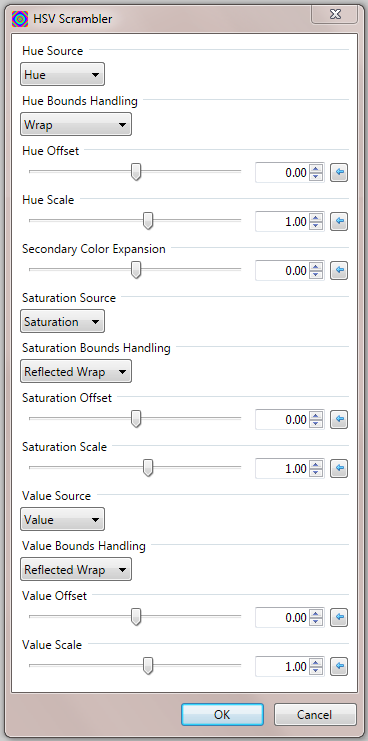Each component has a Source, which can be Hue, Saturation, Value, or One.

The Source component is Offset then scaled by the Scale factor.

Each component also has a choice of Bounds Handling, which can be Wrap, Reflected Wrap, or Clamp. Wrap wraps all values to 0 to 1, Clamp clamps out-of-range values to 0 or 1, and Reflected Wrap maps values up to 1 then backwards down to 0. Reflected Wrap prevents the discontinuities at boundaries for Saturation and Value. It isn't necessary in order to make the Hue continuous.

The Hue has a Secondary Color Expansion control. I thought the secondary color (yellow, cyan, and magenta) bands were sometimes too thin relative to the primary (red, green, and blue) color bands, so I added a control to optionally widen the bands. It can also be used to narrow the secondary bands, if desired. I think 0.5 often works quite well.

Probably the most common choice will be Value for the Hue source, and One for the Saturation and Value sources. This pseudo colors a black and white image.

Here is a quick example: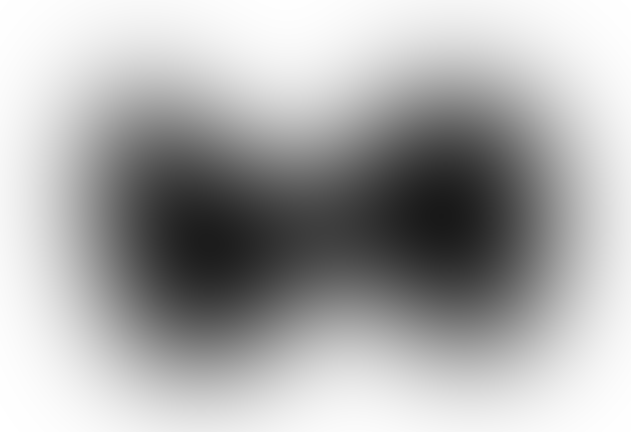After HSV Scrambler: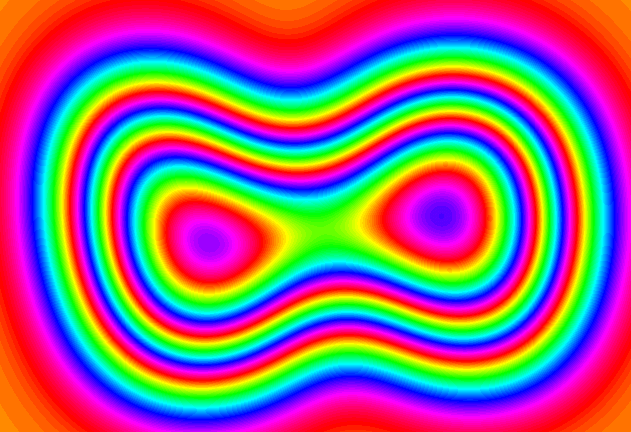Here is the CodeLab code:

Spoiler
```
// Author: MJW
// Name: HSV Scrambler
// Title: HSV Scrambler
// Desc: Scramble the HSV components
// Keywords: scramble HSV color
#region UICode
byte Amount1 = 0; // Hue Source|Hue|Saturation|Value|One
byte Amount2 = 0; // Hue Bounds Handling|Wrap|Reflected Wrap|Clamp
double Amount3 = 0; // [-1,1] Hue Offset
double Amount4 = 1; // [-8,8] Hue Scale
double Amount5 = 0; // [-1,1] Secondary Color Expansion
byte Amount6 = 0; // Saturation Source|Saturation|Value|Hue|One
byte Amount7 = 0; // Saturation Bounds Handling|Reflected Wrap|Clamp|Wrap
double Amount8 = 0; // [-1,1] Saturation Offset
double Amount9 = 1; // [-8,8] Saturation Scale
byte Amount10 = 0; // Value Source|Value|Hue|Saturation|One
byte Amount11 = 0; // Value Bounds Handling|Reflected Wrap|Clamp|Wrap
double Amount12 = 0; // [-1,1] Value Offset
double Amount13 = 1; // [-8,8] Value Scale
#endregion

delegate double getDouble();
delegate double transformDouble(double d);
private transformDouble [] selectBoundsH, selectBoundsSV;

// Here is the main render loop function
void Render(Surface dst, Surface src, Rectangle rect)
{
double offsetH, offsetS, offsetV;
double scaleH, scaleS, scaleV;
double secondaryExpansion;
getDouble getH, getS, getV;
transformDouble boundH, boundS, boundV;
double srcH = 0.0, srcS = 0.0, srcV = 0.0;

// Bounds handling routines.
if (selectBoundsH == null)
{
selectBoundsH = new transformDouble [] {Wrap, ReflectedWrap, Clamp};
selectBoundsSV = new transformDouble [] {ReflectedWrap, Clamp, Wrap};
}
boundH = selectBoundsH[Amount2];
boundS = selectBoundsSV[Amount7];
boundV = selectBoundsSV[Amount11];

// Offsets and scaling factors.
offsetH = Amount3; scaleH = Amount4;
offsetS = Amount8; scaleS = Amount9;
offsetV = Amount12; scaleV = Amount13;

// Amount to expand the secondary colors (yellow, cyan, and magenta).
secondaryExpansion = (Amount5 < 0) ? 0.5 * Amount5 : Amount5;

unsafe
{
// Source of the component.
double one = 1.0;
double *pH = (Amount1 < 2) ?
((Amount1 == 0) ? &srcH : &srcS) : ((Amount1 == 2) ? &srcV : &one);
double *pS = (Amount6 < 2) ?
((Amount6 == 0) ? &srcS : &srcV) : ((Amount6 == 2) ? &srcH : &one);
double *pV = (Amount10 < 2) ?
((Amount10 == 0) ? &srcV : &srcH) : ((Amount10 == 2) ? &srcS : &one);

// Rendering loop.
for (int y = rect.Top; y < rect.Bottom; y++)
{
if (IsCancelRequested) return;
for (int x = rect.Left; x < rect.Right; x++)
{
ColorBgra CurrentPixel = src[x, y];
byte a = CurrentPixel.A;
RGBtoHSV(CurrentPixel, out srcH, out srcS, out srcV);
double h = boundH(offsetH + scaleH * *pH);
if (secondaryExpansion != 0.0)
double s = boundS(offsetS + scaleS * *pS);
double v = boundV(offsetV + scaleV * *pV);
dst[x, y] = HSVtoRGBA(h, s, v, a);
}
}
}
}

double Clamp(double d)
{
return (d < 0.0) ? 0.0 : ((d > 1.0) ? 1.0 : d);
}

double Wrap(double d)
{
return d - Math.Floor(d);
}

double ReflectedWrap(double d)
{
d = Math.Abs(d) % 2.0;
return (d > 1.0) ? 2.0 - d : d;
}

const double oneThird = 1.0 / 3.0;
{
double tripledH = 3.0 * HueConstrain(h);
double frac = tripledH - Math.Floor(tripledH);
double twiceFrac = 2.0 * frac;
tripledH += secondaryExpansion * twiceFrac * (frac - 1.0) * (twiceFrac - 1.0);
return oneThird * tripledH;
}

public ColorBgra HSVtoRGBA(double H, double S, double V, double A)
{
return HSVtoRGBA(H, S, V, (byte)(255 * A + 0.5));
}

public ColorBgra HSVtoRGBA(double H, double S, double V, byte A)
{
byte r, g, b;
HSVtoRGB(H, S, V, out r, out g, out b);
return ColorBgra.FromBgra(b, g, r, (byte)A);
}

public void HSVtoRGB(double H, double S, double V, out byte bR, out byte bG, out byte bB)
{
// Parameters must satisfy the following ranges:
// 0.0 <= H < 1.0
// 0.0 <= S <= 1.0
// 0.0 <= V <= 1.0

// Handle special case of gray (so no Hue) first
if ((S == 0.0) || (V == 0.0))
{
byte x = (byte)(int)(V * 255.0);
bR = x;
bG = x;
bB = x;
return;
}

H = HueConstrain(H);

double R = V, G = V, B = V;
double Hi = Math.Floor(6.0 * H);
double f = 6.0 * H - Hi;
double p = V * (1.0 - S);
double q = V * (1.0 - f * S);
double t = V * (1.0 - (1.0 - f) * S);
if (Hi == 0.0)
{
R = V;
G = t;
B = p;
}
else if (Hi == 1.0)
{
R = q;
G = V;
B = p;
}
else if (Hi == 2.0)
{
R = p;
G = V;
B = t;
}
else if (Hi == 3.0)
{
R = p;
G = q;
B = V;
}
else if (Hi == 4.0)
{
R = t;
G = p;
B = V;
}
else // if (Hi == 5.0)
{
R = V;
G = p;
B = q;
}

int iR = (int)(R * 255.0 + 0.5);
int iG = (int)(G * 255.0 + 0.5);
int iB = (int)(B * 255.0 + 0.5);
bR = (byte)iR;
bG = (byte)iG;
bB = (byte)iB;
}

public void RGBtoHSV(ColorBgra color, out double H, out double S, out double V)
{
RGBtoHSV(color.R, color.G, color.B, out H, out S, out V);
}

public void RGBtoHSV(int R, int G, int B, out double outH, out double outS, out double outV)
{
const double H_UNDEFINED = 0.0;    // Arbitrarily set undefined hue to 0
const double recip6 = 1.0 / 6.0;

// R, G, and B must range from 0 to 255
// Ouput value ranges:
// outH - 0.0 to 1.0
// outS - 0.0 to 1.0
// outV - 0.0 to 1.0

double dR = (double)R / 255.0;
double dG = (double)G / 255.0;
double dB = (double)B / 255.0;
double dmaxRGB = Max3(dR, dG, dB);
double dminRGB = Min3(dR, dG, dB);
double delta = dmaxRGB - dminRGB;

// Set value
outV = dmaxRGB;

// Handle special case of V = 0 (black)
if (dmaxRGB == 0)
{
outH = H_UNDEFINED;
outS = 0.0;
return;
}

// Handle specai case of S = 0 (gray)
outS = delta / dmaxRGB;
if (dmaxRGB == dminRGB)
{
outH = H_UNDEFINED;
return;
}

// Finally, compute hue
if (dR == dmaxRGB)
{
outH = (dG - dB) / delta;
}
else if (dG == dmaxRGB)
{
outH = 2.0 + (dB - dR) / delta;
}
else //if (dB == dmaxRGB)
{
outH = 4.0 + (dR - dG) / delta;
}

outH *= recip6;
outH = HueConstrain(outH);
}

public double Max3(double x, double y, double z)
{
return (x > y) ? ((x > z) ? x : z) : ((y > z) ? y : z);
}

public double Min3(double x, double y, double z)
{
return (x < y) ? ((x < z) ? x : z) : ((y < z) ? y : z);
}

public double HueConstrain(double MyHue)
{
// Makes sure that 0.0 <= MyAngle < 1.0
// Wraps around the value if its outside this range
while (MyHue >= 1.0)
{
MyHue -= 1.0;
}
while (MyHue < 0.0)
{
MyHue += 1.0;
}
return MyHue;
}

```

Here is the plugin: HsvScrambler.zip

EDIT: Version 1.1. Add control to optionally widen the secondary Hue color bands. Change scale ranges from 10 to 8. Modify method of  fetching source components  (using "unsafe" pointers suggested by midora). Use different selection method for sources, and slightly revise method for selecting bounding delegates. Move bounding selection control next to component selection. It makes less sense logically, but I believe it makes the interface clearer.

•4
##### Share on other sites

I hate bothering one more time with my compilation difficulties, but I'm experiencing what seems to be a number of syntax errors at the following lines

```getH = new getDouble [] {(() => inH), (() => inS), (() => inV), (() => 1.0)}[Amount1];
getS = new getDouble [] {(() => inS), (() => inV), (() => inH), (() => 1.0)}[Amount5];
getV = new getDouble [] {(() => inV), (() => inH), (() => inS), (() => 1.0)}[Amount9];
```

Ideas, alternatives, clues, etc. would be highly appreciated. As it's widely known, I'm under the 3.5.11 version of CodeLabEdited by Maximilian
##### Share on other sites

It's probably not really CodeLab that's the problem. The Lambda expressions are part of C#. I thought they went back pretty far and would work with the older versions. In any case, I'm not really sure that's such a great way to do it, anyway. I was just playing around with an alternative to using a switch statement. Lambda expressions really just delegates, but with the important advantage  (for this situation) of being able to access local variables. I'll probably add an additional feature very soon, and when I do, I'll try to use a different approach to fetching these variables that will work with older versions. Most likely I'll assign the input HSV components to an array, and set the proper indices to get the desired component. Or, if someone has a better method, I'd appreciate hearing it. What I don't want to do, just because it's annoyingly long-winded, is to use a switch at the point the input components are accessed. I'm not too fond of using conditional assignments, either, though given that there are four options, it'd be pretty efficient.

##### Share on other sites

Thanks to delegates and lambdas you created a quite compact way to implement the algorithm.

For me that's absolute fine but for sure if someone has no idea about delegates and lambdas it may be difficult.

The mapping defined by a Lambda to a local variable in c# is like to define a pointer in C to a local.

So if you set the Render function to unsafe then you could rewrite the getH/S/V functions in the following way.

double dOne = 1.0;
double *getH = new double*[] {&inH, &inS, &inV, &dOne} [Amount1];

...

double h = boundH(offsetH + scaleH * *getH);

Unsafe allows you to optimze the access to src and dst via pointers.

BTW:For me it's confusing that you prefix the out variables with 'in' ;-)##### Share on other sites

midora, that sounds like a good method, though I generally avoid using unsafe. I'm not sure why I avoid unsafe, since I used C for many years; perhaps because of the scary name.

The "in" part actually makes sense to me, though I'm willing to change it if it makes the code clearer. They're called "in" because they represent the input values that get reassigned (scrambled) to produce the output values. Maybe srcH, srcS, srcV would be clearer.

I'll probably change the original assignments of delegates, indices, or pointers to use two-level conditional assignments instead of the array method. The array method is great if the table can be reused, but probably doesn't make sense when new arrays have to be created for each roi. The conditional assignment method isn't particularly elegant, but is likely more efficient. The bounding delegate selection could be done from tables that are just initialized once, if the array pointers are null, and I may do that.

Edited by MJW
##### Share on other sites

I made some changes to HSV Scrambler. It's now version 1.1. Edit notes at bottom of original comment.

##### Share on other sites

Thanks MJW! Little toys are nice to play with too.##### Share on other sites

Could a monitor please move this thread over to Plugins -- Publishing Only? That's where I intended to put it. This plugin may not be that polished, but I consider it to be officially published.

##### Share on other sites

Fresh out of monitors. Will an Administrator do?•1ebook: Mastering Paint.NET | resources: Plugin Index | Stereogram Tut | proud supporter of Codelab
plugins: EER's Plugin Pack | Planetoid | StickMan | WhichSymbol+ | Dr Scott's Markup Renderer | CSV Filetype | dwarf horde plugins: Plugin Browser | ShapeMaker

##### Share on other sites

Thank you, Ego! I can never remember the correct terminology, but it apparently achieved the desired result.

##### Share on other sites

And since I've been monitoring () this thread, I hereby append a version compatible with the good old Paint.NET 3.5.11, suitable for the hard-of-updating(least I can do for getting MJW into coding trouble)

HSV Scrambler 1.1 for PdN 3.5.11.zip

##### Share on other sites

I'm not sure whether the below-posted quickies will look like a far-fetched application of the plugin, but I've been experimenting with what seems to be an acceptable method to give a photo a phantasmic appearance (or phantasmically cartoony, or something like that).

For the following example I create what I have dubbed (tentatively) a phantasmic mask by doing an HSV Scramble on a copy of the original picture, then inverting colors, then Linocut, and finally setting the blend mode to color burn. To give it an even more phantasmal appearance I apply some jitter to the phantasmic mask. The intermediate step of inverting colors may be unnecessary, but I left it as is because it gave me the outcome I was expecting. Optionally, one way to make the result look even paler (or ghostlier) is repeating the HSV Scramble + invert colors operation on another dupe of the original image placed above the phantasmic mask layer with its blend mode set to overlay: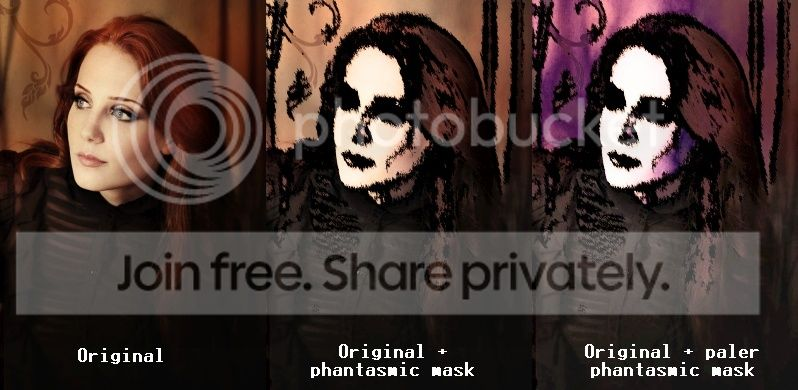And for this other example I've followed basically the same steps, but doing a Motion Blur instead of a Jitter: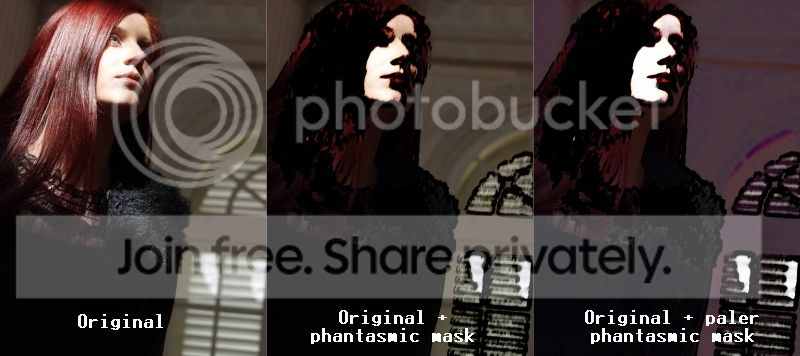Here are the settings I've used for both HSV Scrambler and Linocut:Of course everything may be perfected. What I've shown here is merely the result of quick plays that haven't been much refined.

•1
##### Share on other sites

Of course everything may be perfected. What I've shown here is merely the result of quick plays that haven't been much refined.

Very original. The work worthy of imitation (emulation).

Edited by ReMake
##### Share on other sites

Thank you, ReMake! As you can see, I've found a way to put your Linocut plugin to a different kind of use##### Share on other sites

Thank you, ReMake! As you can see, I've found a way to put your Linocut plugin to a different kind of useThank You.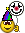Edited by ReMake
##### Share on other sites

That's quite an imaginative use of the HSV Scrambler, Maximilian. The plugin can also be used to produce effects that resemble Solarization and similar sort of metallic looks. They're kind of interesting, though it seems to be quite sensitive to JPEG noise. One of these days I hope to find something really useful it can do.

##### Share on other sites

Thank you, MJW! I'll continue to explore its possibilities. What I posted above came out of the blue but I'm pretty sure that further uses remain to be discovered## Join the conversation

You can post now and register later. If you have an account, sign in now to post with your account.×   Pasted as rich text.   Paste as plain text instead

Only 75 emoji are allowed.

×   Your previous content has been restored.   Clear editor

×   You cannot paste images directly. Upload or insert images from URL.

×
×